Next: Complex Velocity Up: Two-Dimensional Potential Flow Previous: Cauchy-Riemann Relations

# Complex Velocity Potential

Equations (6.17)-(6.18) are identical to Equations (5.21)-(5.22). This suggests that the real and imaginary parts of a well-behaved function of the complex variable can be interpreted as the velocity potential and stream function, respectively, of some two-dimensional, irrotational, incompressible flow pattern.

For instance, suppose that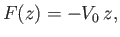(6.23)

where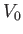is real. It follows that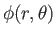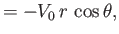(6.24)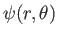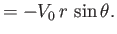(6.25)

It can be seen, by comparison with the analysis of Section 5.4, that the complex velocity potential (6.23) corresponds to uniform flow of speeddirected along the-axis. Furthermore, as is easily demonstrated, the complex velocity potential associated with uniform flow of speedwhose direction subtends a (counter-clockwise) angle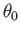with the-axis is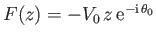.

Suppose that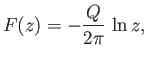(6.26)

where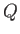is real. Because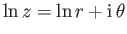(Riley 1974), it follows that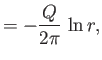(6.27)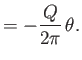(6.28)

Thus, according to the analysis of Section 5.5, the complex velocity potential (6.26) corresponds to the flow pattern of a line source, of strength, located at the origin. (See Figure 5.3.) As a simple generalization of this result, the complex potential of a line source, of strength, located at the point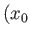,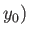, is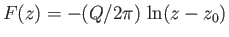, where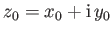. It can be seen, from Equation (6.26), that the complex velocity potential of a line source is singular at the location of the source.

Suppose that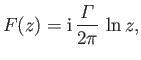(6.29)

where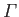is real. It follows that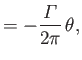(6.30)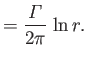(6.31)

Thus, according to the analysis of Section 5.6, the complex velocity potential (6.29) corresponds to the flow pattern of a vortex filament of intensitylocated at the origin. (See Figure 5.5.) As a simple generalization of this result, the complex potential of a vortex filament, of intensity, located at the point,, is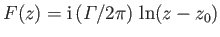, where. According to Equation (6.29), the complex velocity potential of a vortex filament is singular at the location of the filament.

Suppose, finally, that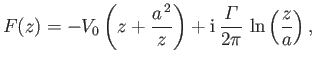(6.32)

where,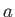, and, are real. It follows that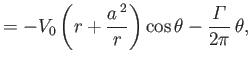(6.33)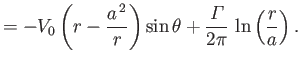(6.34)

Thus, according to the analysis of Section 5.8, the complex velocity potential (6.32) corresponds to uniform flow of unperturbed speed, running parallel to the-axis, around an impenetrable circular cylinder of radius, centered on the origin. (See Figures 5.6, 5.7, and 5.8.) Here,is the circulation of the flow about the cylinder. It can be seen that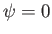on the surface of the cylinder (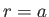), which ensures that the normal velocity is zero on this surface (because the surface corresponds to a streamline), as must be the case if the cylinder is impenetrable.Next: Complex Velocity Up: Two-Dimensional Potential Flow Previous: Cauchy-Riemann Relations
Richard Fitzpatrick 2016-03-31